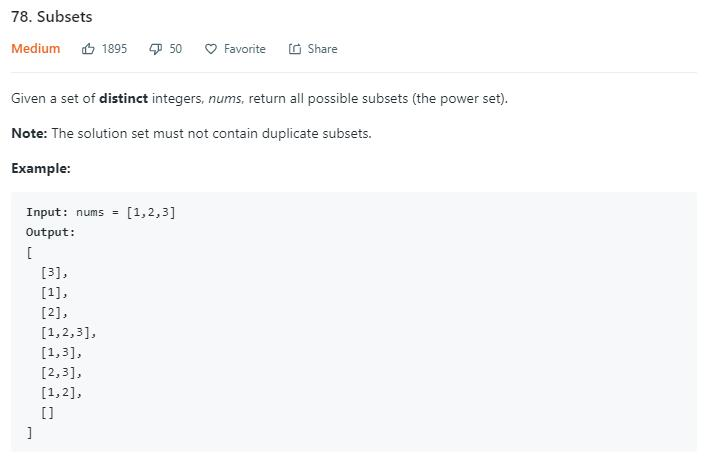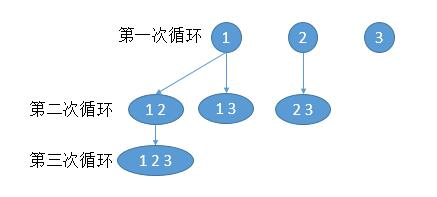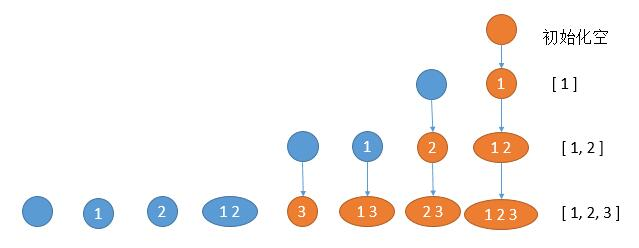# 题目描述（中等难度）# 解法一 迭代一

77 题解法三一个思想，想找出数组长度 1 的所有解，然后再在长度为 1 的所有解上加 1 个数字变成长度为 2 的所有解，同样的直到 n。public List<List<Integer>> subsets(int[] nums) {
List<List<Integer>> res = new ArrayList<List<Integer>>();
List<List<Integer>> ans = new ArrayList<List<Integer>>();
int n = nums.length;
// 第一层循环，子数组长度从 1 到 n
for (int i = 1; i <= n; i++) {
// 第二层循环，遍历上次的所有结果
List<List<Integer>> tmp = new ArrayList<List<Integer>>();
for (List<Integer> list : res) {
// 第三次循环，对每个结果进行扩展
for (int m = 0; m < n; m++) {
//只添加比末尾数字大的数字，防止重复
if (list.size() > 0 && list.get(list.size() - 1) >= nums[m])
continue;
List<Integer> newList = new ArrayList<Integer>(list);
}
}
res = tmp;
}
return ans;
}


# 解法二 迭代法2public List<List<Integer>> subsets(int[] nums) {
List<List<Integer>> ans = new ArrayList<>();
for(int i = 0;i<nums.length;i++){
List<List<Integer>> ans_tmp = new ArrayList<>();
//遍历之前的所有结果
for(List<Integer> list : ans){
List<Integer> tmp = new ArrayList<>(list);
}
}
return ans;
}


# 解法三 回溯法

public List<List<Integer>> subsets(int[] nums) {
List<List<Integer>> ans = new ArrayList<>();
getAns(nums, 0, new ArrayList<>(), ans);
return ans;
}

private void getAns(int[] nums, int start, ArrayList<Integer> temp, List<List<Integer>> ans) {
for (int i = start; i < nums.length; i++) {
getAns(nums, i + 1, temp, ans);
temp.remove(temp.size() - 1);
}
}


# 解法四 位操作

1 2 3
0 0 0 -> [     ]
0 0 1 -> [    3]
0 1 0 -> [  2  ]
0 1 1 -> [  2 3]
1 0 0 -> [1    ]
1 0 1 -> [1   3]
1 1 0 -> [1 2  ]
1 1 1 -> [1 2 3]


public List<List<Integer>> subsets(int[] nums) {
List<List<Integer>> ans = new ArrayList<>();
int bit_nums = nums.length;
int ans_nums = 1 << bit_nums; //执行 2 的 n 次方
for (int i = 0; i < ans_nums; i++) {
List<Integer> tmp = new ArrayList<>();
int count = 0; //记录当前对应数组的哪一位
int i_copy = i; //用来移位
while (i_copy != 0) {
if ((i_copy & 1) == 1) { //判断当前位是否是 1
}
count++;
i_copy = i_copy >> 1;//右移一位
}